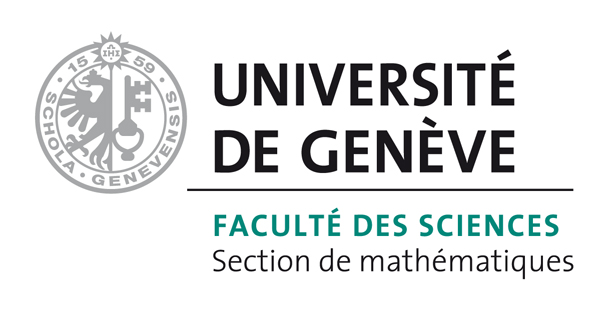# Bart Vandereycken## A Riemannian geometry with complete geodesics for the set of positive semidefinite matrices of fixed rank

by , ,

#### Abstract:

We present a homogeneous space geometry for the manifold of symmetric positive semidefinite matrices of fixed rank. The total space is a connected part of the general linear group endowed with its natural right-invariant metric and the metric on the homogeneous space is chosen such that the quotient space is the image of a Riemannian submersion from the total space. As a result, we obtain complete geodesics that are the image of certain geodesics on the general linear group. We derive in addition an efficient closed-form expression for these geodesics. Furthermore, we isometrically embed the abstract quotient space into the space of real matrices. This allows us to interpret the vector fields, the metric and the geodesics in terms of concrete matrices. Finally, we compare our geometry with some other geometries in the literature. In particular, we show how other geodesics and their approximations relate to ours.

#### Reference:

B. Vandereycken, P.-A. Absil, S. Vandewalle, "A Riemannian geometry with complete geodesics for the set of positive semidefinite matrices of fixed rank", In IMA J. Numer. Anal., vol. 33, no. 2, pp. 481-514, 2012.

#### Bibtex Entry:

@article{VandereyckenAV_2012,
Abstract = {We present a homogeneous space geometry for the manifold of symmetric positive semidefinite matrices of fixed rank. The total space is a connected part of the general linear group endowed with its natural right-invariant metric and the metric on the homogeneous space is chosen such that the quotient space is the image of a Riemannian submersion from the total space. As a result, we obtain complete geodesics that are the image of certain geodesics on the general linear group. We derive in addition an efficient closed-form expression for these geodesics. Furthermore, we isometrically embed the abstract quotient space into the space of real matrices. This allows us to interpret the vector fields, the metric and the geodesics in terms of concrete matrices. Finally, we compare our geometry with some other geometries in the literature. In particular, we show how other geodesics and their approximations relate to ours.},
Author = {Vandereycken, B. and Absil, P.-A. and Vandewalle, S.},
Doi = {10.1093/imanum/drs006},
Journal = {IMA J. Numer. Anal.},
Number = {2},
Pages = {481--514},
Pdf = {http://www.unige.ch/math/vandereycken/papers/preprint_VandereyckenAV_2012.pdf},
Title = {A {R}iemannian geometry with complete geodesics for the set of positive semidefinite matrices of fixed rank},
Volume = {33},
Year = {2012}}